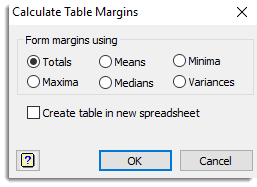1. Home
2. Create Table Margins

# Create Table Margins

Use this to create a margin for a table spreadsheet. The margins can be formed by various summary statistics.## Form margins using

 Totals Creates the margins using the total of the values in the table. Mean Creates the margins using the mean of the values in the table. Minima Creates the margins using the minimum of the values in the table. Maxima Creates the margins using the maximum of the values in the table. Median Creates the margins using the median of the values in the table. Variance Creates the margins using the variance of the values in the table.

Note the summary statistic is formed based on the information within the cells and does not take account of any weights or the number of observations used in calculating the values.

## Create table in new spreadsheet

When selected the table will be duplicated in a new spreadsheet with a new name. If this option has not been selected the margin will be added to the table in the current spreadsheet.